# Test: Memory Management & Virtual Memory- 1

## 30 Questions MCQ Test GATE Computer Science Engineering(CSE) 2023 Mock Test Series | Test: Memory Management & Virtual Memory- 1

Description
Attempt Test: Memory Management & Virtual Memory- 1 | 30 questions in 90 minutes | Mock test for Computer Science Engineering (CSE) preparation | Free important questions MCQ to study GATE Computer Science Engineering(CSE) 2023 Mock Test Series for Computer Science Engineering (CSE) Exam | Download free PDF with solutions
QUESTION: 1

### Which of the following page replacement algorithms suffers from Belady’s anomaly?

Solution:

Belady’s anomaly proves that it is possible to have more page faults when increasing the number of page frames while using the First in First Out (FIFO) page replacement algorithm. See the example given on Wiki Page.

QUESTION: 2

### What is the swap space in the disk used for?

Solution:

Swap space is typically used to store process data. See this for more details.

QUESTION: 3

### Increasing the RAM of a computer typically improves performance because:

Solution:

When there is more RAM, there would be more mapped virtual pages in physical memory, hence fewer page faults. A page fault causes performance degradation as the page has to be loaded from secondary device.

QUESTION: 4

A computer system supports 32-bit virtual addresses as well as 32-bit physical addresses. Since the virtual address space is of the same size as the physical address space, the operating system designers decide to get rid of the virtual memory entirely. Which one of the following is true?

Solution:

For supporting virtual memory, special hardware support is needed from Memory Management Unit. Since operating system designers decide to get rid of the virtual memory entirely, hardware support for memory management is no longer needed

QUESTION: 5

A CPU generates 32-bit virtual addresses. The page size is 4 KB. The processor has a translation look-aside buffer (TLB) which can hold a total of 128 page table entries and is 4-way set associative. The minimum size of the TLB tag is:

Solution:

Size of a page = 4KB = 2^12 Total number of bits needed to address a page frame = 32 - 12 = 20 If there are ‘n’ cache lines in a set, the cache placement is called n-way set associative. Since TLB is 4 way set associative and can hold total 128 (2^7) page table entries, number of sets in cache = 2^7/4 = 2^5. So 5 bits are needed to address a set, and 15 (20 - 5) bits are needed for tag.

QUESTION: 6

Virtual memory is

Solution:

Virtual memory is illusion of large main memory.

QUESTION: 7

Page fault occurs when

Solution:

Page fault occurs when a requested page is mapped in virtual address space but not present in memory.

QUESTION: 8

Thrashing occurs when

Solution:

Thrashing occurs processes on system require more memory than it has. If processes do not have “enough” pages, the pagefault rate is very high. This leads to: – low CPU utilization – operating system spends most of its time swapping to disk The above situation is called thrashing

QUESTION: 9

A computer uses 46–bit virtual address, 32–bit physical address, and a three–level paged page table organization. The page table base register stores the base address of the first–level table (T1), which occupies exactly one page. Each entry of T1 stores the base address of a page of the second–level table (T2). Each entry of T2 stores the base address of a page of the third–level table (T3). Each entry of T3 stores a page table entry (PTE). The PTE is 32 bits in size. The processor used in the computer has a 1 MB 16 way set associative virtually indexed physically tagged cache. The cache block size is 64 bytes. What is the size of a page in KB in this computer?

Solution:

Let the page size is of 'x' bits
Size of T1 = 2 ^ x bytes
(This is because T1 occupies exactly one page)
Now, number of entries in T1 = (2^x) / 4
(This is because each page table entry is 32 bits or 4 bytes in size)
Number of entries in T1 = Number of second level page tables
(Because each I-level page table entry stores the base address of page of II-level page table)
Total size of second level page tables = ((2^x) / 4) * (2^x)
Similarly, number of entries in II-level page tables = Number of III level page tables = ((2^x) / 4) * ((2^x) / 4)
Total size of third level page tables = ((2^x) / 4) * ((2^x) / 4) * (2^x)
Similarly, total number of entries (pages) in all III-level
page tables = ((2^x) / 4) * ((2^x) / 4) * ((2^x) / 4)
= 2^(3x - 6)
Size of virtual memory = 2^46 Number of pages in virtual memory = (2^46) / (2^x) = 2^(46 - x)
Total number the pages in the III-level page tables = Number of pages in virtual memory
2^(3x - 6) = 2^(46 - x)
3x - 6 = 46 - x
4x = 52
x = 13
That means, page size is of 13 bits or Page size = 2^13 bytes = 8 KB

QUESTION: 10

Consider data given in the above question. What is the minimum number of page colours needed to guarantee that no two synonyms map to different sets in the processor cache of this computer?

Solution:

1 MB 16-way set associative virtually indexed physically tagged cache(VIPT). The cache block size is 64 bytes.
No of blocks is 2^20/2^6 = 2^14.
No of sets is 2^14/2^4 = 2^10.

VA(46)
+-------------------------------+
tag(30) , Set(10) , block offset(6)
+-------------------------------+

In VIPT if the no. of bits of page offset = (Set+block offset) then only one page color is sufficient.
but we need 8 colors because the number bits where the cache set index and physical page number over lap is 3 so 2^3 page colors is required.(option c is ans).

QUESTION: 11

Consider the virtual page reference string 1, 2, 3, 2, 4, 1, 3, 2, 4, 1 On a demand paged virtual memory system running on a computer system that main memory size of 3 pages frames which are initially empty. Let LRU, FIFO and OPTIMAL denote the number of page faults under the corresponding page replacements policy. Then

Solution:

First In First Out (FIFO) This is the simplest page replacement algorithm. In this algorithm, operating system keeps track of all pages in the memory in a queue; oldest page is in the front of the queue. When a page needs to be replaced page in the front of the queue is selected for removal. Optimal Page replacement: in this algorithm, pages are replaced which are not used for the longest duration of time in the future. Least Recently Used (LRU) In this algorithm page will be replaced which is least recently used. Solution: the virtual page reference string is 1, 2, 3, 2, 4, 1, 3, 2, 4, 1 size of main memory pages frames is 3. For FIFO: total no of page faults are 6 (depicted in bold and italic)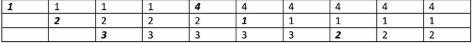For optimal: total no of page faults are 5 (depicted in bold and italic)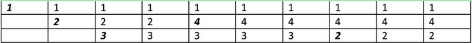For LRU: total no of page faults are 9 (depicted in bold and italic)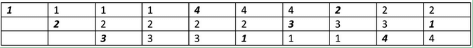The Optimal will be 5, FIFO 6 and LRU 9. so, OPTIMAL < FIFO < LRU option (B) is correct answer.

QUESTION: 12

Let the page fault service time be 10ms in a computer with average memory access time being 20ns. If one page fault is generated for every 10^6 memory accesses, what is the effective access time for the memory?

Solution:

Let P be the page fault rate
Effective Memory Access Time = p * (page fault service time) + (1 - p) * (Memory access time)
= ( 1/(10^6) )* 10 * (10^6) ns + (1 - 1/(10^6)) * 20 ns
= 30 ns (approx)

QUESTION: 13

A system uses FIFO policy for page replacement. It has 4 page frames with no pages loaded to begin with. The system first accesses 100 distinct pages in some order and then accesses the same 100 pages but now in the reverse order. How many page faults will occur?

Solution:
QUESTION: 14

In which one of the following page replacement policies, Belady’s anomaly may occur?

Solution:

Belady’s anomaly proves that it is possible to have more page faults when increasing the number of page frames while using the First in First Out (FIFO) page replacement algorithm. See the wiki page for an example of increasing page faults with number of page frames.

QUESTION: 15

The essential content(s) in each entry of a page table is / are

Solution:

A page table entry must contain Page frame number. Virtual page number is typically used as index in page table to get the corresponding page frame number. See this for details.

QUESTION: 16

A multilevel page table is preferred in comparison to a single level page table for translating virtual address to physical address because

Solution:

The size of page table may become too big (See this) to fit in contiguous space. That is why page tables are typically divided in levels.

QUESTION: 17

A processor uses 36 bit physical addresses and 32 bit virtual addresses, with a page frame size of 4 Kbytes. Each page table entry is of size 4 bytes. A three level page table is used for virtual to physical address translation, where the virtual address is used as follows
• Bits 30-31 are used to index into the first level page table
• Bits 21-29 are used to index into the second level page table
• Bits 12-20 are used to index into the third level page table, and
• Bits 0-11 are used as offset within the page.
The number of bits required for addressing the next level page table (or page frame) in the page table entry of the first, second and third level page tables are respectively.

Solution:

Virtual address size = 32 bits Physical address size = 36 bits Physical memory size = 2^36 bytes Page frame size = 4K bytes = 2^12 bytes No. of bits for offset (or number of bits required for accessing location within a page frame) = 12. No. of bits required to access physical memory frame = 36 - 12 = 24 So in third level of page table, 24 bits are required to access an entry. 9 bits of virtual address are used to access second level page table entry and size of pages in second level is 4 bytes. So size of second level page table is (2^9)*4 = 2^11 bytes. It means there are (2^36)/(2^11) possible locations to store this page table. Therefore the second page table requires 25 bits to address it. Similarly, the third page table needs 25 bits to address it.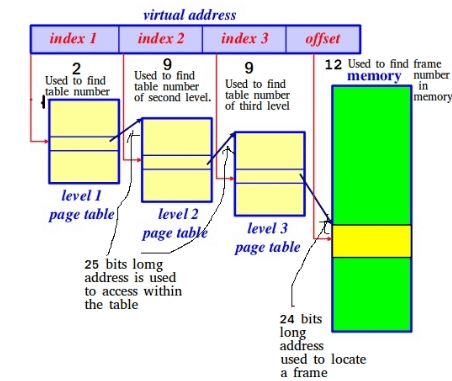QUESTION: 18

A virtual memory system uses First In First Out (FIFO) page replacement policy and allocates a fixed number of frames to a process. Consider the following statements:

P: Increasing the number of page frames allocated to a process sometimes increases the page fault rate.
Q: Some programs do not exhibit locality of reference.

Q. Which one of the following is TRUE?

Solution:

First In First Out Page Replacement Algorithms: This is the simplest page replacement algorithm. In this algorithm, operating system keeps track of all pages in the memory in a queue, oldest page is in the front of the queue. When a page needs to be replaced page in the front of the queue is selected for removal. FIFO Page replacement algorithms suffers from Belady’s anomaly : Belady’s anomaly states that it is possible to have more page faults when increasing the number of page frames. Solution:
Statement P: Increasing the number of page frames allocated to a process sometimes increases the page fault rate. Correct, as FIFO page replacement algorithm suffers from belady’s anomaly which states above statement.
Statement Q: Some programs do not exhibit locality of reference. Correct, Locality often occurs because code contains loops that tend to reference arrays or other data structures by indices. So we can write a program does not contain loop and do not exhibit locality of reference. So, both statement P and Q are correct but Q is not the reason for P as Belady’s Anomaly occurs for some specific patterns of page references.

QUESTION: 19

A process has been allocated 3 page frames. Assume that none of the pages of the process are available in the memory initially. The process makes the following sequence of page references (reference string): 1, 2, 1, 3, 7, 4, 5, 6, 3, 1 If optimal page replacement policy is used, how many page faults occur for the above reference string?

Solution:

Optimal replacement policy looks forward in time to see which frame to replace on a page fault. 1 23    -> 1,2,3 //page faults 173      ->7 143  ->4 153 -> 5 163  -> 6 Total=7

QUESTION: 20

Consider the data given in above question. Least Recently Used (LRU) page replacement policy is a practical approximation to optimal page replacement. For the above reference string, how many more page faults occur with LRU than with the optimal page replacement policy?

Solution:

LRU replacement policy: The page that is least recently used is being Replaced. Given String:   1, 2, 1, 3, 7, 4, 5, 6, 3, 1 123  // 1 ,2, 3 //page faults 173 ->7 473 ->4 453 ->5 456 ->6 356 ->3 316 ->1 Total 9, In optimal Replacement total page faults=7 Therefore 2 more page faults  Answer is C

QUESTION: 21

Assume that there are 3 page frames which are initially empty. If the page reference string is 1, 2, 3, 4, 2, 1, 5, 3, 2, 4, 6, the number of page faults using the optimal replacement policy is__________.

Solution:

In optimal page replacement replacement policy, we replace the place which is not used for longest duration in future.

Given three page frames.
Reference string is 1, 2, 3, 4, 2, 1, 5, 3, 2, 4, 6 Initially, there are three page faults and entries are 1 2 3 Page 4 causes a page fault and replaces 3 (3 is the longest distant in future), entries become 1 2 4
Total page faults = 3+1 = 4
Pages 2 and 1 don't cause any fault.

5 causes a page fault and replaces 1, entries become 5 2 4
Total page faults = 4 + 1 = 5
3 causes a page fault and replaces 1, entries become 3 2 4
Total page faults = 5 + 1 = 6
3, 2 and 4 don't cause any page fault. 6 causes a page fault.
Total page faults = 6 + 1 = 7

QUESTION: 22

A computer has twenty physical page frames which contain pages numbered 101 through 120. Now a program accesses the pages numbered 1, 2, …, 100 in that order, and repeats the access sequence THRICE. Which one of the following page replacement policies experiences the same number of page faults as the optimal page replacement policy for this program?

Solution:

The optimal page replacement algorithm swaps out the page whose next use will occur farthest in the future. In the given question, the computer has 20 page frames and initially page frames are filled with pages numbered from 101 to 120. Then program accesses the pages numbered 1, 2, …, 100 in that order, and repeats the access sequence THRICE. The first 20 accesses to pages from 1 to 20 would definitely cause page fault. When 21st is accessed, there is another page fault. The page swapped out would be 20 because 20 is going to be accessed farthest in future. When 22nd is accessed, 21st is going to go out as it is going to be the farthest in future. The above optimal page replacement algorithm actually works as most recently used in this case. As a side note, the first 100 would cause 100 page faults, next 100 would cause 81 page faults (1 to 19 would never be removed), the last 100 would also cause 81 page faults.

QUESTION: 23

A system uses 3 page frames for storing process pages in main memory. It uses the Least Recently Used (LRU) page replacement policy. Assume that all the page frames are initially empty. What is the total number of page faults that will occur while processing the page reference string given below? 4, 7, 6, 1, 7, 6, 1, 2, 7, 2

Solution:

What is a Page fault ? An interrupt that occurs when a program requests data that is not currently in real memory. The interrupt triggers the operating system to fetch the data from a virtual memory and load it into RAM. Now, 4, 7, 6, 1, 7, 6, 1, 2, 7, 2 is the reference string, you can think of it as data requests made by a program. Now the system uses 3 page frames for storing process pages in main memory. It uses the Least Recently Used (LRU) page replacement policy.
[ ] - Initially page frames are empty.i.e. no process pages in main memory.
 - Now 4 is brought into 1st frame (1st page fault)
Explanation: Process page 4 was requested by the program, but it was not in the main memory(in form of page frames),which resulted in a page fault, after that process page 4 was brought in the main memory by the operating system.
[4 7] - Now 7 is brought into 2nd frame (2nd page fault) - Same explanation.
[4 7 6] - Now 6 is brought into 3rd frame (3rd page fault)
[1 7 6] - Now 1 is brought into 1st frame, as 1st frame was least recently used(4th page fault).

After this 7, 6 and 1 are were already present in the frames hence no replacements in pages.

[1 2 6] - Now 2 is brought into 2nd frame, as 2nd frame was least recently used(5th page fault).
[1 2 7] -Now 7 is brought into 3rd frame, as 3rd frame was least recently used(6th page fault).

Hence, total number of page faults(also called pf) are 6. Therefore, C is the answer.

QUESTION: 24

Consider a paging hardware with a TLB. Assume that the entire page table and all the pages are in the physical memory. It takes 10 milliseconds to search the TLB and 80 milliseconds to access the physical memory. If the TLB hit ratio is 0.6, the effective memory access time (in milliseconds) is _________.

Solution:

QUESTION: 25

The memory access time is 1 nanosecond for a read operation with a hit in cache, 5 nanoseconds for a read operation with a miss in cache, 2 nanoseconds for a write operation with a hit in cache and 10 nanoseconds for a write operation with a miss in cache. Execution of a sequence of instructions involves 100 instruction fetch operations, 60 memory operand read operations and 40 memory operand write operations. The cache hit-ratio is 0.9. The average memory access time (in nanoseconds) in executing the sequence of instructions is __________.

Solution:

The question is to find the time taken for, "100 fetch operation and 60 operand red operations and 40 memory operand write operations"/"total number of instructions".
Total number of instructions= 100+60+40 =200 Time taken for 100 fetch operations(fetch =read) = 100*((0.9*1)+(0.1*5)) // 1 corresponds to time taken for read // when there is cache hit = 140 ns //0.9 is cache hit rate
Time taken for 60 read operations = 60*((0.9*1)+(0.1*5)) = 84ns
Time taken for 40 write operations = 40*((0.9*2)+(0.1*10)) = 112 ns
// Here 2 and 10 the time taken for write when there is cache
// hit and no cahce hit respectively So,the total time taken for 200 operations is = 140+84+112 = 336ns
Average time taken = time taken per operation = 336/200 = 1.68 ns

QUESTION: 26

A CPU generates 32-bit virtual addresses. The page size is 4 KB. The processor has a translation look-aside buffer (TLB) which can hold a total of 128 page table entries and is 4-way set associative. The minimum size of the TLB tag is:

Solution:

Virtual Memory would not be very effective if every memory address had to be translated by looking up the associated physical page in memory. The solution is to cache the recent translations in a Translation Lookaside Buffer (TLB). A TLB has a fixed number of slots that contain page table entries, which map virtual addresses to physical addresses.
Solution Size of a page = 4KB = 2^12 means 12 offset bits CPU generates 32-bit virtual addresses Total number of bits needed to address a page frame = 32 - 12 = 20 If there are ‘n’ cache lines in a set, the cache placement is called n-way set associative. Since TLB is 4 way set associative and can hold total 128 (2^7) page table entries, number of sets in cache = 2^7/4 = 2^5. So 5 bits are needed to address a set, and 15 (20 - 5) bits are needed for tag. Option (C) is the correct answer.

QUESTION: 27

A computer system supports 32-bit virtual addresses as well as 32-bit physical addresses. Since the virtual address space is of the same size as the physical address space, the operating system designers decide to get rid of the virtual memory entirely. Which one of the following is true?

Solution:
QUESTION: 28

The minimum number of page frames that must be allocated to a running process in a virtual memory environment is determined by

Solution:

There are two important tasks in virtual memory management: a page-replacement strategy and a frame-allocation strategy. Frame allocation strategy says gives the idea of minimum number of frames which should be allocated. The absolute minimum number of frames that a process must be allocated is dependent on system architecture, and corresponds to the number of pages that could be touched by a single (machine) instruction. So, it is instruction set architecture i.e. option (A) is correct answer.

QUESTION: 29

Consider a system with a two-level paging scheme in which a regular memory access takes 150 nanoseconds, and servicing a page fault takes 8 milliseconds. An average instruction takes 100 nanoseconds of CPU time, and two memory accesses. The TLB hit ratio is 90%, and the page fault rate is one in every 10,000 instructions. What is the effective average instruction execution time?

Solution: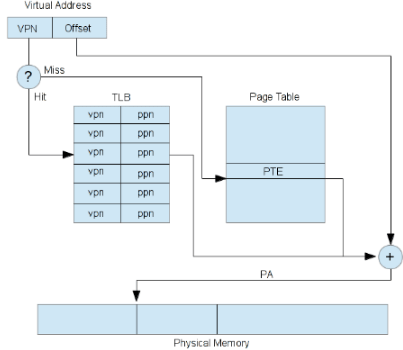Figure : Translation Lookaside Buffer

As shown in figure, to find frame number for corresponding page number, at first TLB (Translation Lookaside Buffer) is checked whether it has that desired page number- frame number pair entry or not, if yes then it’s TLB hit otherwise it’s TLB miss. In case of miss the page number is searched into page table. In two-level paging scheme, the memory is referred two times to obtain corresponding frame number.

• If a virtual address has no valid entry in the page table, then any attempt by your pro- gram to access that virtual address will cause a page fault to occur .In case of page fault, the required frame is brought in main memory from secondary memory,time taken to service the page fault is called page fault service time.

• We have to caculate average execution time(EXE), lets suppose average memory ac- cess time to fetch is M, then EXE = 100ns + 2*150 (two memory references to fetch instruction) + M ...1

• Now we have to calculate average memory access time M, since page fault is 1 in 10,000 instruction and then M = (1 − 1/104 )(M EM ) + (1/104) ∗ 8ms ...2

• Where MEM is memory access time when page is present in memory. Now we calcu- late MEM MEM = .9(TLB Access Time)+.1(TLB Access Time+2*150ns)

• Here TLB Acess Time is not given lets assume it 0. So MEM=.9(0)+.1(300ns) =30ns , put MEM value in equation(2). M = (1 − 1/104 )(30ns) + (1/104 ) ∗ 8ms = 830ns

• Put this M's value in equation(1), EXE=100ns+300ns+830ns=1230ns , so Ans is option(4).

QUESTION: 30

In a system with 32 bit virtual addresses and 1 KB page size, use of one-level page tables for virtual to physical address translation is not practical because of

Solution:Use Code STAYHOME200 and get INR 200 additional OFF Use Coupon Code